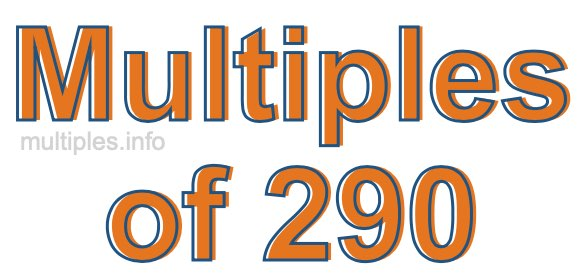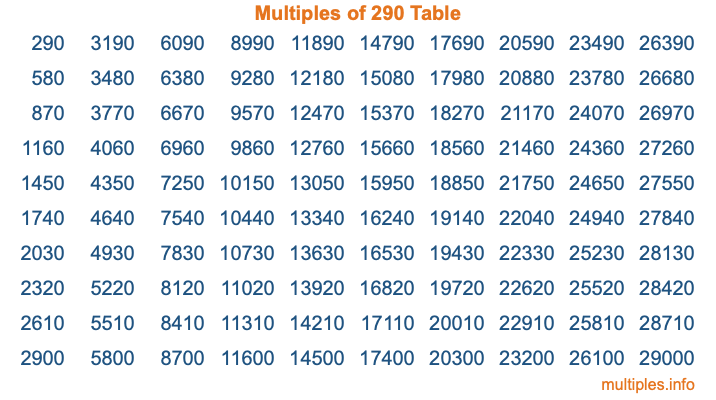Multiples of 290Welcome to the Multiples of 290 page. Here we will first teach you everything you will ever need to know about the multiples of 290, and then give you a study guide summary of everything we taught you to make sure you remember it all. Use this page to look up facts and learn information about the multiples of 290. This page will make you a multiples of two hundred ninety expert!

Definition of Multiples of 290
Multiples of 290 are all the numbers that when divided by 290 equal an integer. Each of the multiples of 290 are called a multiple. A multiple of 290 is created by multiplying 290 by an integer.

Therefore, to create a list of multiples of 290, you start with 1 multiplied by 290, then 2 multiplied by 290, then 3 multiplied by 290, and so on for as long as you want. Thus, the list of the first five multiples of 290 is 290, 580, 870, 1160, and 1450. To see a larger list of multiples of 290, see the printable image of Multiples of 290 further down on this page. We also have a category where you can choose any nth multiple of 290.

Multiples of 290 Checker
The Multiples of 290 Checker below checks to see if any number of your choice is a multiple of 290. In other words, it checks to see if there is any number (integer) that when multiplied by 290 will equal your number. To do that, we divide your number by 290. If the the quotient is an integer, then your number is a multiple of 290.

Is  a multiple of 290?

Least Common Multiple of 290 and ...
A Least Common Multiple (LCM) is the lowest multiple that two or more numbers have in common. This is also called the smallest common multiple or lowest common multiple and is useful to know when you are adding our subtracting fractions. Enter one or more numbers below (290 is already entered) to find the LCM.

Check out our LCM Calculator if you need more details about the Least Common Multiple or if you need the LCM for different numbers for adding and subtraction fractions.

nth Multiple of 290
As we stated above, 290 is the first multiple of 290, 580 is the second multiple of 290, 870 is the third multiple of 290, and so on. Enter a number below to find the nth multiple of 290.

th multiple of 290

Multiples of 290 vs Factors of 290
290 is a multiple of 290 and a factor of 290, but that is where the similarities end. All postive multiples of 290 are 290 or greater than 290. All positive factors of 290 are 290 or less than 290.

Below is the beginning list of multiples of 290 and the factors of 290 so you can compare:

Multiples of 290: 290, 580, 870, 1160, 1450, etc.

Factors of 290: 1, 2, 5, 10, 29, 58, 145, 290

As you can see, the multiples of 290 are all the numbers that you can divide by 290 to get a whole number. The factors of 290, on the other hand, are all the whole numbers that you can multiply by another whole number to get 290.

It's also interesting to note that if a number (x) is a factor of 290, then 290 will also be a multiple of that number (x).

Multiples of 290 vs Divisors of 290
The divisors of 290 are all the integers that 290 can be divided by evenly. Below is a list of the divisors of 290.

Divisors of 290: 1, 2, 5, 10, 29, 58, 145, 290

The interesting thing to note here is that if you take any multiple of 290 and divide it by a divisor of 290, you will see that the quotient is an integer.

Multiples of 290 Table
Below is an image of the first 100 multiples of 290 in a table. The table is in chronological order, column by column. The first column has the first ten multiples of 290, the second column has the next ten multiples of 290, and so on.The Multiples of 290 Table is also referred to as the 290 Times Table or Times Table of 290. You are welcome to print out our table for your studies.

Negative Multiples of 290
Although not often discussed or needed in math, it is worth mentioning that you can make a list of negative multiples of 290 by multiplying 290 by -1, then by -2, then by -3, and so on, to get the following list of negative multiples of 290:

-290, -580, -870, -1160, -1450, etc.

Multiples of 290 Summary
Below is a summary of important Multiples of 290 facts that we have discussed on this page. To retain the knowledge on this page, we recommend that you read through the summary and explain to yourself or a study partner why they hold true.

There are an infinite number of multiples of 290.

A multiple of 290 divided by 290 will equal a whole number.

290 divided by a factor of 290 equals a divisor of 290.

The nth multiple of 290 is n times 290.

The largest factor of 290 is equal to the first positive multiple of 290.

290 is a multiple of every factor of 290.

290 is a multiple of 290.

A multiple of 290 divided by a divisor of 290 equals an integer.

290 divided by a divisor of 290 equals a factor of 290.

Any integer times 290 will equal a multiple of 290.

Multiples of a Number
Here you can get the multiples of another number, all with the same attention to detail as we did for multiples of 290 on this page.

Multiples of
Multiples of 291
Did you find our page about multiples of two hundred ninety educational? Do you want more knowledge? Check out the multiples of the next number on our list!